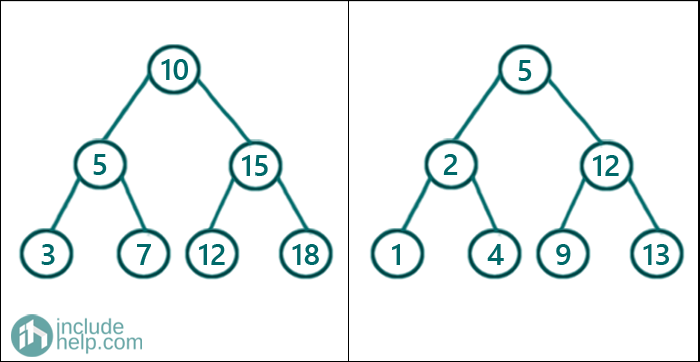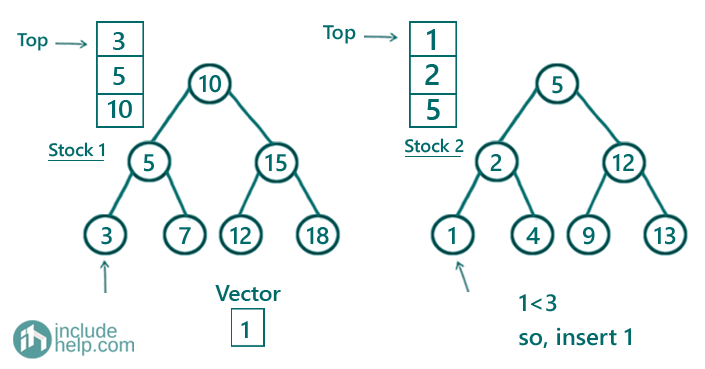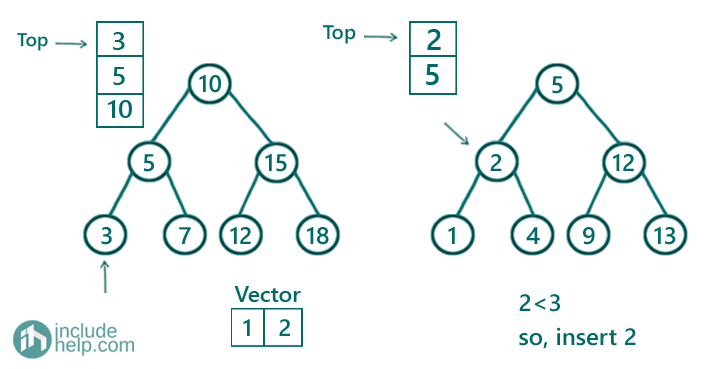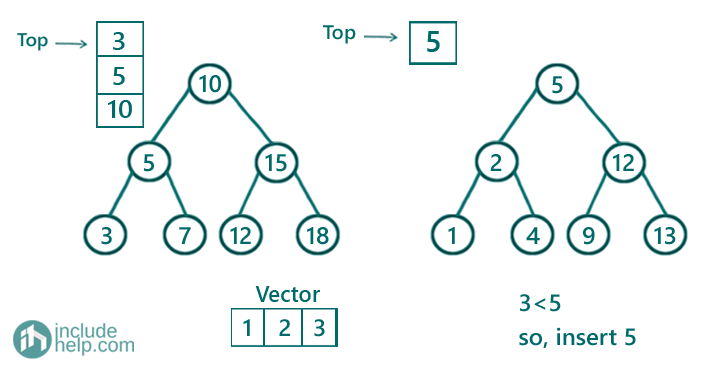# Merge two Binary Search Trees set 2 (limited space)

In this article, we are going to see how to merge two binary search trees efficiently?
Submitted by Radib Kar, on November 07, 2020

Prerequisite: Inorder traversal without recursion

In the last post, we saw the naïve solution where we used to set data structure as additional space. But here, we will do without any additional data structure to store the elements in order. Here, we will use iterative inorder traversal for both the trees to solve this.After merging the above two trees result would be:

1 2 3 4 5 5 7 9 10 12 12 13 15 18

Here, we will do the iterative inorder traversal pretty similarly as we did in our article Count Number of pairs from two different BSTs whose sum is equal to X.

So the idea is to do iterative inorder traversal and compare b/w the currently pointed nodes. Whichever node is small will be inserted first and the pointer will be incremented. Then we will continue to traverse again. The detailed algorithm is below:

1. First, declare a vector that will store the merged inorder traversal(final result).
2. Declare two stacks st1 & st2 which will do the iterative inorder traversal.
3. While(true){
1. Go as left as possible and keep pushing all nodes into the stacks while going left. Do the same for both the trees.
2. If both stacks are empty that means there are no more nodes left
3. If any of the stacks are empty that means there is only one tree left to be traversed, so add the traversal left for that node to the vector
4. Otherwise, both stacks are not empty and in that case,
1. Say cur1 & cur2 is the top nodes from the respective stacks.
If cur1 value is less than cur2 value then insert cur1 value to the vector and pop out cur1. Set root1 to be the right child of cur1.
Else If cur2 value is less than cur2 value then insert cur2 value to the vector and pop out cur2. Set root2 to be the right child of cur2.
Else both have the same value so insert both to the vector & pop out from the stacks. Root1 & root2 will be set as the right child of cur1 & cur2 respectively.
End While

Below is the C++ implementation of the above algorithm.

```#include <bits/stdc++.h>
using namespace std;

// tree node is defined
class TreeNode {
public:
int val;
TreeNode* left;
TreeNode* right;
TreeNode(int data)
{
val = data;
left = NULL;
right = NULL;
}
};

void print(vector<int>& arr)
{
for (auto it : arr) {
cout << it << " ";
}
cout << endl;
}

vector<int> getAllElements(TreeNode* root1, TreeNode* root2)
{
vector<int> myvec;
stack<TreeNode *> st1, st2;
TreeNode *cur1, *cur2;

while (true) {
while (root1) {
st1.push(root1);
root1 = root1->left;
}
while (root2) {
st2.push(root2);
root2 = root2->left;
}
if (st1.empty() && st2.empty())
break;

if (st1.empty())
cur1 = NULL;
else
cur1 = st1.top();

if (st2.empty())
cur2 = NULL;
else
cur2 = st2.top();
if (cur1 && cur2) {
if (cur1->val < cur2->val) {
myvec.push_back(cur1->val);
st1.pop();
root1 = cur1->right;
}
else if (cur1->val > cur2->val) {
myvec.push_back(cur2->val);
st2.pop();
root2 = cur2->right;
}
else {
myvec.push_back(cur1->val);
myvec.push_back(cur2->val);
st1.pop();
st2.pop();
root1 = cur1->right;
root2 = cur2->right;
}
}
else {
if (cur1) {
myvec.push_back(cur1->val);
st1.pop();
root1 = cur1->right;
}
else {
myvec.push_back(cur2->val);
st2.pop();
root2 = cur2->right;
}
}
}
return myvec;
}

int main()
{
//trees built like the example
TreeNode* root1 = new TreeNode(10);

root1->left = new TreeNode(5);
root1->right = new TreeNode(15);
root1->left->left = new TreeNode(3);
root1->left->right = new TreeNode(7);
root1->right->left = new TreeNode(12);
root1->right->right = new TreeNode(18);

TreeNode* root2 = new TreeNode(5);
root2->left = new TreeNode(2);
root2->right = new TreeNode(12);
root2->left->left = new TreeNode(1);
root2->left->right = new TreeNode(4);
root2->right->left = new TreeNode(9);
root2->right->right = new TreeNode(13);

vector<int> mergedArr = getAllElements(root1, root2);

cout << "After merging two binary search trees, inorder traversal of the merged tree is:\n";
print(mergedArr);

return 0;
}
```

Output:

```After merging two binary search trees, inorder traversal of the merged tree is:
1 2 3 4 5 5 7 9 10 12 12 13 15 18
```

Dry run:

We will show initial few steps here to illustrate how it's working.

1. So first we go as left as possible for both the trees and keep pushing nodes into the stacks that come while going towards left. So current stack tops are 3 & 1 respectively, since 1 is less than 3, insert 1 to the vector and pop it out. root1 & stack1 will remain the same. Root2 would be the right child of 1 which is NULL.2. Since both roots are NULL, it won't add any more element in the corresponding stacks. Now stack tops are respectively 3 & 2. Again 2 is smaller than 3, thus we will insert 2 into the vector. root1 & stack1 will remain the same and stack2 will pop out 2. Root2 will be 2->right which is NULL.3. Since both roots are NULL, won't insert any nodes into the stacks. Current stack tops are 3 & 5 respectively. So we will insert 3 and pop 3 out of stack 1. Rott1 will be 3->right which is NULL. Stack2 and root2 will remain unchanged.
In this way, we can proceed until both the stacks become empty. You can carry on the dry run if you still need to learn more. Finally we will have the vector [1 2 3 4 5 5 7 9 10 12 12 13 15 18]So, this is a much better implementation which uses no extra space to store the traversals. Though we have used stack space that is equivalent to the stack space needed for recursive inorder traversal (due to recursive calls).

Languages: » C » C++ » C++ STL » Java » Data Structure » C#.Net » Android » Kotlin » SQL
Web Technologies: » PHP » Python » JavaScript » CSS » Ajax » Node.js » Web programming/HTML
Solved programs: » C » C++ » DS » Java » C#
Aptitude que. & ans.: » C » C++ » Java » DBMS
Interview que. & ans.: » C » Embedded C » Java » SEO » HR
CS Subjects: » CS Basics » O.S. » Networks » DBMS » Embedded Systems » Cloud Computing
» Machine learning » CS Organizations » Linux » DOS
More: » Articles » Puzzles » News/Updates